Tamil Nadu Board of Secondary EducationSSLC (English Medium) Class 7th

# Tamil Nadu Board Samacheer Kalvi solutions for Class 7th Mathematics Term 3 Answers Guide chapter 4 - Geometry [Latest edition]

#### Chapters## Chapter 4: Geometry

Exercise 4.1Exercise 4.2Exercise 4.3
Exercise 4.1 [Pages 84 - 87]

### Tamil Nadu Board Samacheer Kalvi solutions for Class 7th Mathematics Term 3 Answers Guide Chapter 4 GeometryExercise 4.1 [Pages 84 - 87]

Exercise 4.1 | Q 1. (i) | Page 84

Find the new position point using the translation given

2→, 4↓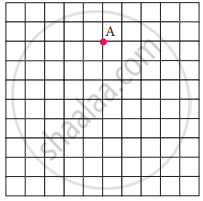Exercise 4.1 | Q 1. (ii) | Page 84

Find the new position point using the translation given

6↑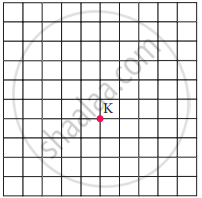Exercise 4.1 | Q 1. (iii) | Page 84

Find the new position point using the translation given

3←, 5↓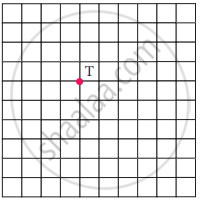Exercise 4.1 | Q 1. (iv) | Page 84

Find the new position point using the translation given

4→, 3↑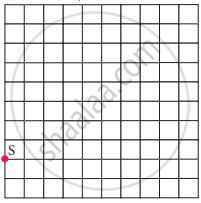Exercise 4.1 | Q 2. (i) | Page 84

How is the pre-image translated to the image?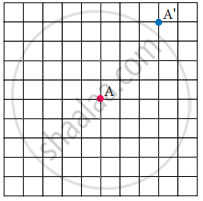Exercise 4.1 | Q 2. (ii) | Page 84

How is the pre-image translated to the image?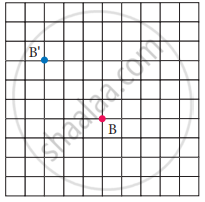Exercise 4.1 | Q 2. (iii) | Page 84

How is the pre-image translated to the image?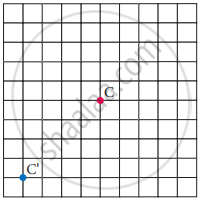Exercise 4.1 | Q 2. (iv) | Page 84

How is the pre-image translated to the image?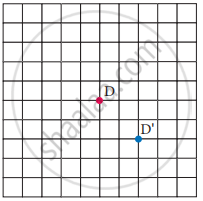Exercise 4.1 | Q 3. (i) | Page 85

Find the image of the given triangle with given translation

4↑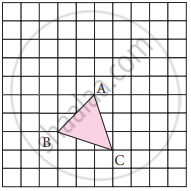Exercise 4.1 | Q 3. (ii) | Page 85

Find the image of the given triangle with given translation

6→ 3↓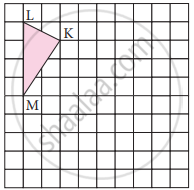Exercise 4.1 | Q 3. (iii) | Page 85

Find the image of the given triangle with given translation

5← 4↓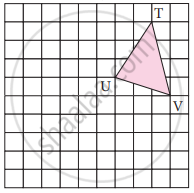Exercise 4.1 | Q 3. (iv) | Page 85

Find the image of the given triangle with given translation

4→ 3↑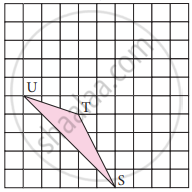Exercise 4.1 | Q 4. (i) | Page 85

Reflect the shape with given line of reflection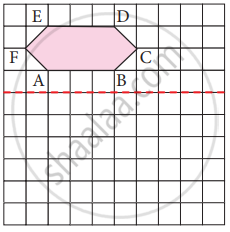Exercise 4.1 | Q 4. (ii) | Page 85

Reflect the shape with given line of reflection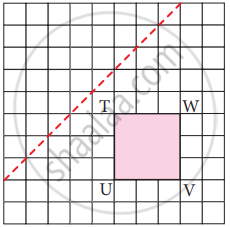Exercise 4.1 | Q 4. (iii) | Page 85

Reflect the shape with given line of reflection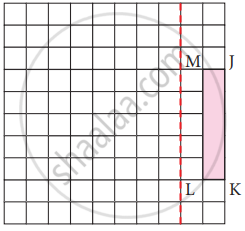Exercise 4.1 | Q 4. (iv) | Page 85

Reflect the shape with given line of reflection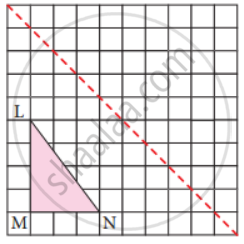Exercise 4.1 | Q 4. (v) | Page 85

Reflect the shape with given line of reflection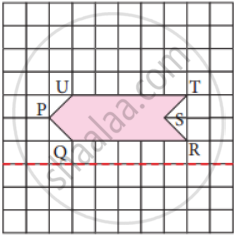Exercise 4.1 | Q 4. (vi) | Page 85

Reflect the shape with given line of reflection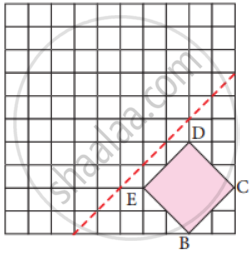Exercise 4.1 | Q 5. (i) | Page 85

Reflect the shape in the following picture with given line of reflection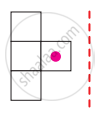Exercise 4.1 | Q 5. (ii) | Page 85

Reflect the shape in the following picture with given line of reflection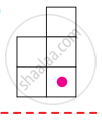Exercise 4.1 | Q 5. (iii) | Page 85

Reflect the shape in the following picture with given line of reflection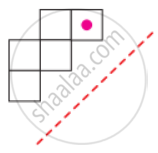Exercise 4.1 | Q 6. (i) | Page 85

Rotate the preimage as directed about the green point

90° clockwise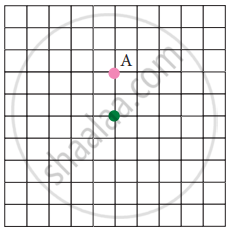Exercise 4.1 | Q 6. (ii) | Page 85

Rotate the preimage as directed about the green point

180°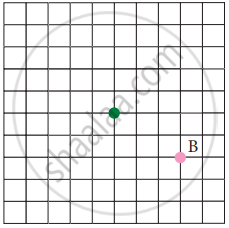Exercise 4.1 | Q 6. (iii) | Page 85

Rotate the preimage as directed about the green point

270° counter clockwise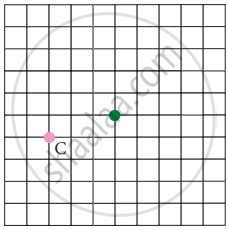Exercise 4.1 | Q 6. (iv) | Page 86

Rotate the preimage as directed about the green point

90° counter clockwise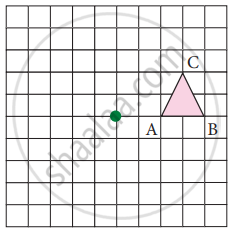Exercise 4.1 | Q 6. (v) | Page 86

Rotate the preimage as directed about the green point

90° clockwise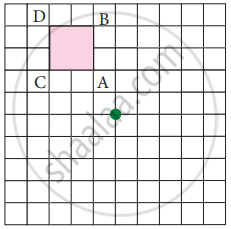Exercise 4.1 | Q 6. (vi) | Page 86

Rotate the preimage as directed about the green point

180°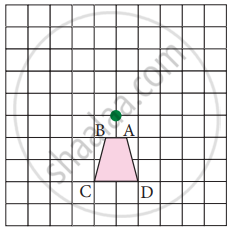Exercise 4.1 | Q 7 | Page 86

Identify the transformation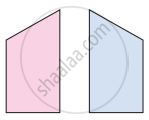Exercise 4.1 | Q 8 | Page 86

Identify the transformation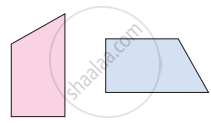Exercise 4.1 | Q 9 | Page 86

Identify the transformation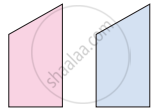Exercise 4.1 | Q 10 | Page 86

A pool of fish translates from point F to point D.
a. Describe the translation of the pool of fish.
b. Can the fishing boat make the same translation? Explain.
c. Describe a translation the fishing boat could make to get to point D.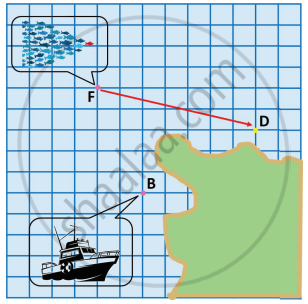Exercise 4.1 | Q 11. (i) | Page 86

Name the transformation that will map footprint A onto the indicated footprint.

Footprint B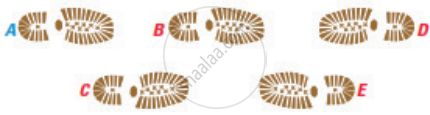Exercise 4.1 | Q 11. (ii) | Page 86

Name the transformation that will map footprint A onto the indicated footprint.

Footprint CExercise 4.1 | Q 11. (iii) | Page 86

Name the transformation that will map footprint A onto the indicated footprint.

Footprint DExercise 4.1 | Q 11. (iv) | Page 86

Name the transformation that will map footprint A onto the indicated footprint.

Footprint EExercise 4.1 | Q 12. (i) | Page 86

In given diagram, the blue figure is an image of the pink figure.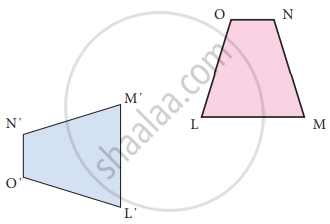Choose an angle or a vertex from the preimage and name its image

Exercise 4.1 | Q 12. (ii) | Page 86

In given diagram, the blue figure is an image of the pink figure.List all pairs of corresponding sides

Exercise 4.1 | Q 13 | Page 87

In the diagram at the right, the green figure is a translation image of the pink figure. Write a coordinate rule that describes the translation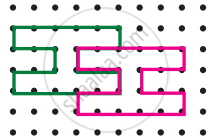#### Objective Type Questions

Exercise 4.1 | Q 14 | Page 87

A _______ is a turn about a point

• Translation

• Rotation

• Reflection

• Glide Reflection

Exercise 4.1 | Q 15 | Page 87

A _______ is a flip over a line

• Translation

• Rotation

• Reflection

• Glide Reflection

Exercise 4.1 | Q 16 | Page 87

A _______ is a slide, move without turning or flipping the shape

• Translation

• Rotation

• Reflection

• Glide Reflection

Exercise 4.1 | Q 17 | Page 87

The transformation used in the picture is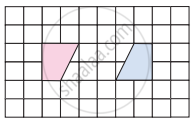• Translation

• Rotation

• Reflection

• Glide Reflection

Exercise 4.1 | Q 18 | Page 87

The transformation used in the picture is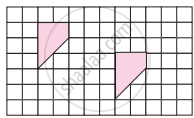• Translation

• Rotation

• Reflection

• Glide Reflection

Exercise 4.1 | Q 19 | Page 87

You must rotate the puzzle piece 270° clockwise about the point P to fit it into a puzzle. Which piece fits in the puzzle as shown?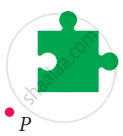•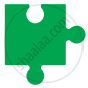•••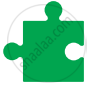Exercise 4.2 [Page 89]

### Tamil Nadu Board Samacheer Kalvi solutions for Class 7th Mathematics Term 3 Answers Guide Chapter 4 GeometryExercise 4.2 [Page 89]

Exercise 4.2 | Q 1. (i) | Page 89

Draw circle for the following measurements of radius(r)/diameters(d)

r = 4 cm

Exercise 4.2 | Q 1. (ii) | Page 89

Draw circle for the following measurements of radius(r)/diameters(d)

d = 12 cm

Exercise 4.2 | Q 1. (iii) | Page 89

Draw circle for the following measurements of radius(r)/diameters(d)

r = 3.5 cm

Exercise 4.2 | Q 1. (iv) | Page 89

Draw circle for the following measurements of radius(r)/diameters(d)

r = 6.5 cm

Exercise 4.2 | Q 1. (v) | Page 89

Draw circle for the following measurements of radius(r)/diameters(d)

d = 6 cm

Exercise 4.2 | Q 2. (i) | Page 89

Draw concentric circle for the following measurements of radii/diameters. Find out the width of the circular ring.

r = 3 cm and r = 5 cm

Exercise 4.2 | Q 2. (ii) | Page 89

Draw concentric circle for the following measurements of radii/diameters. Find out the width of the circular ring.

r = 3.5 cm and r = 6.5 cm

Exercise 4.2 | Q 2. (iii) | Page 89

Draw concentric circle for the following measurements of radii/diameters. Find out the width of the circular ring.

d = 6.4 cm and d = 11.6 cm

Exercise 4.2 | Q 2. (iv) | Page 89

Draw concentric circle for the following measurements of radii/diameters. Find out the width of the circular ring.

r = 5 cm and r = 7.5 cm

Exercise 4.2 | Q 2. (v) | Page 89

Draw concentric circle for the following measurements of radii/diameters. Find out the width of the circular ring.

d = 6.2 cm and r = 6.2 cm

Exercise 4.2 | Q 2. (vi) | Page 89

Draw concentric circle for the following measurements of radii/diameters. Find out the width of the circular ring.

r = 7.1 cm and d = 12 cm

Exercise 4.3 [Pages 90 - 91]

### Tamil Nadu Board Samacheer Kalvi solutions for Class 7th Mathematics Term 3 Answers Guide Chapter 4 GeometryExercise 4.3 [Pages 90 - 91]

#### Miscellaneous Practice problems

Exercise 4.3 | Q 1 | Page 90

The bishop, in given picture of chess board, can move diagonally along dark squares. Describe the translations of the bishop after two moves as shown in the figure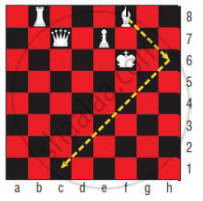Exercise 4.3 | Q 2 | Page 90

Write a possible translation for each of chess piece for a single move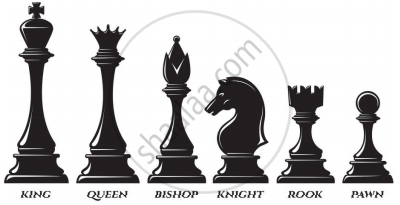Exercise 4.3 | Q 3 | Page 90

Referring the graphic given, answer the following questions. Each bar of the category is made up of boy-girl-boy unit,

(i) Which categories show a boy-girl-boy unit that is translation within the bar?

(ii) Which categories show a boy-girl-boy unit that is reflected within the bar?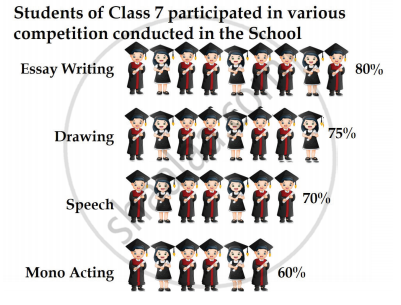Exercise 4.3 | Q 4 | Page 90

Given figure is a floor design in which the length of the small red equilateral triangle is 30 cm. All the triangles and hexagons are regular. Describe the translations in cm, represented by the (i) yellow line (ii) black line (iii) blue line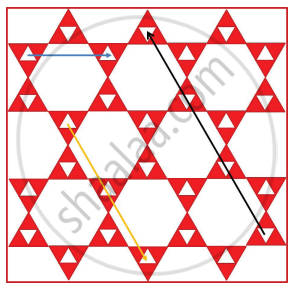Exercise 4.3 | Q 5. (i) | Page 90

Describe the transformation involved in the following pair of figure (letter). Write translation, reflection or rotation.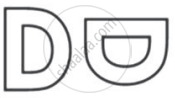Exercise 4.3 | Q 5. (ii) | Page 90

Describe the transformation involved in the following pair of figure (letter). Write translation, reflection or rotation.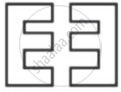Exercise 4.3 | Q 5. (iii) | Page 90

Describe the transformation involved in the following pair of figure (letter). Write translation, reflection or rotation.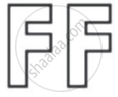Exercise 4.3 | Q 5. (iv) | Page 90

Describe the transformation involved in the following pair of figure (letter). Write translation, reflection or rotation.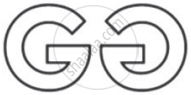Exercise 4.3 | Q 5. (v) | Page 90

Describe the transformation involved in the following pair of figure (letter). Write translation, reflection or rotation.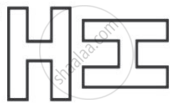Exercise 4.3 | Q 5. (vi) | Page 90

Describe the transformation involved in the following pair of figure (letter). Write translation, reflection or rotation.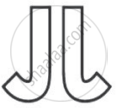Exercise 4.3 | Q 5. (vii) | Page 90

Describe the transformation involved in the following pair of figure (letter). Write translation, reflection or rotation.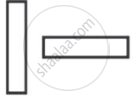Exercise 4.3 | Q 5. (viii) | Page 90

Describe the transformation involved in the following pair of figure (letter). Write translation, reflection or rotation.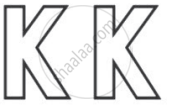#### Challenge Problems

Exercise 4.3 | Q 6 | Page 91

In chess, a knight can move only in an L-shaped pattern:
● two vertical squares, then one horizontal square
● two horizontal squares, then one vertical square
● one vertical square, then two horizontal squares
● one horizontal square, then two vertical squares
Write a series of translations to move the knight from g8 to g5 [at most two moves].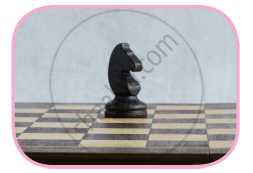Exercise 4.3 | Q 7. (i) | Page 91

The pink shape is congruent to blue shape. Describe a sequence of transformations in which the blue shape is the image of pink shape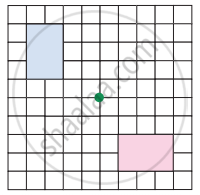Exercise 4.3 | Q 7. (ii) | Page 91

The pink shape is congruent to blue shape. Describe a sequence of transformations in which the blue shape is the image of pink shape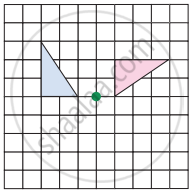Exercise 4.3 | Q 8. (i) | Page 91

Draw the translation of the shape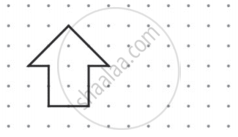Exercise 4.3 | Q 8. (ii) | Page 91

Draw the reflection of the shape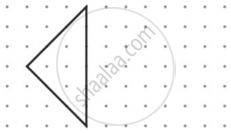Exercise 4.3 | Q 8. (iii) | Page 91

Draw the Rotation of the shape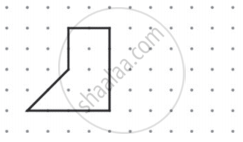Exercise 4.3 | Q 9 | Page 91

Draw concentric circles given that radius of inner circle is 4.5 cm and width of circular ring is 2.5 cm

Exercise 4.3 | Q 10 | Page 91

Draw concentric circles given that radius of outer circle is 5.3 cm and width of circular ring is 1.8 cm

## Chapter 4: Geometry

Exercise 4.1Exercise 4.2Exercise 4.3## Tamil Nadu Board Samacheer Kalvi solutions for Class 7th Mathematics Term 3 Answers Guide chapter 4 - Geometry

Tamil Nadu Board Samacheer Kalvi solutions for Class 7th Mathematics Term 3 Answers Guide chapter 4 (Geometry) include all questions with solution and detail explanation. This will clear students doubts about any question and improve application skills while preparing for board exams. The detailed, step-by-step solutions will help you understand the concepts better and clear your confusions, if any. Shaalaa.com has the Tamil Nadu Board of Secondary Education Class 7th Mathematics Term 3 Answers Guide solutions in a manner that help students grasp basic concepts better and faster.

Further, we at Shaalaa.com provide such solutions so that students can prepare for written exams. Tamil Nadu Board Samacheer Kalvi textbook solutions can be a core help for self-study and acts as a perfect self-help guidance for students.

Concepts covered in Class 7th Mathematics Term 3 Answers Guide chapter 4 Geometry are Concept of Lines Symmetry, Concept of Reflection Symmetry, Concept of Reflection Symmetry, Translational Symmetry, Concept of Symmetry, Symmetry Through Transformations, Concept of Circle - Centre, Radius, Diameter, Arc, Sector, Chord, Segment, Semicircle, Circumference, Interior and Exterior, Concentric Circles, Construction of a Circle When Its Radius is Known, Construction of Concentric Circles.

Using Tamil Nadu Board Samacheer Kalvi Class 7th solutions Geometry exercise by students are an easy way to prepare for the exams, as they involve solutions arranged chapter-wise also page wise. The questions involved in Tamil Nadu Board Samacheer Kalvi Solutions are important questions that can be asked in the final exam. Maximum students of Tamil Nadu Board of Secondary Education Class 7th prefer Tamil Nadu Board Samacheer Kalvi Textbook Solutions to score more in exam.

Get the free view of chapter 4 Geometry Class 7th extra questions for Class 7th Mathematics Term 3 Answers Guide and can use Shaalaa.com to keep it handy for your exam preparation